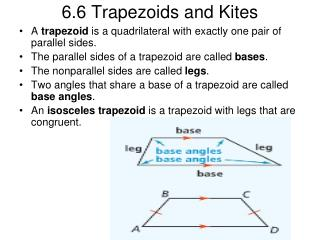DownloadDownload Presentation6.6 Trapezoids and Kites

# 6.6 Trapezoids and Kites

Download Presentation## 6.6 Trapezoids and Kites

- - - - - - - - - - - - - - - - - - - - - - - - - - - E N D - - - - - - - - - - - - - - - - - - - - - - - - - - -
##### Presentation Transcript

1. 6.6 Trapezoids and Kites • A trapezoid is a quadrilateral with exactly one pair of parallel sides. • The parallel sides of a trapezoid are called bases. • The nonparallel sides are called legs. • Two angles that share a base of a trapezoid are called base angles. • An isosceles trapezoid is a trapezoid with legs that are congruent.

2. Theorem 6.19 • If a quadrilateral is an isosceles trapezoid, then each pair of base angles is congruent.

3. Finding Angle Measures in Trapezoids • CDEF is an isosceles trapezoid and the measure of angle C = 65. What are the measures of angle D, angle E, and angle F?

4. Theorem 6.20 • If a quadrilateral is an isosceles trapezoid, then its diagonals are congruent.

5. Midsegment of a Trapezoid • The midsegment of a trapezoid is the segment that joins the midpoints of its legs. • Is parallel to the bases. • The length of the midsegment is half the sum of the lengths of the bases.

6. Kites • A kite is a quadrilateral with two pairs of consecutive sides congruent and no opposite sides congruent.

7. Theorem 6.22 • If a quadrilateral is a kite, then its diagonals are perpendicular.

8. Finding Angle Measures in Kites • Quadrilateral DEFG is a kite. What are the measures of angle 1, angle 2, and angle 3?

9. Concept Summary

10. More Practice!!!!! • Homework – Textbook p. 394 – 395 #7 – 36 ALL.